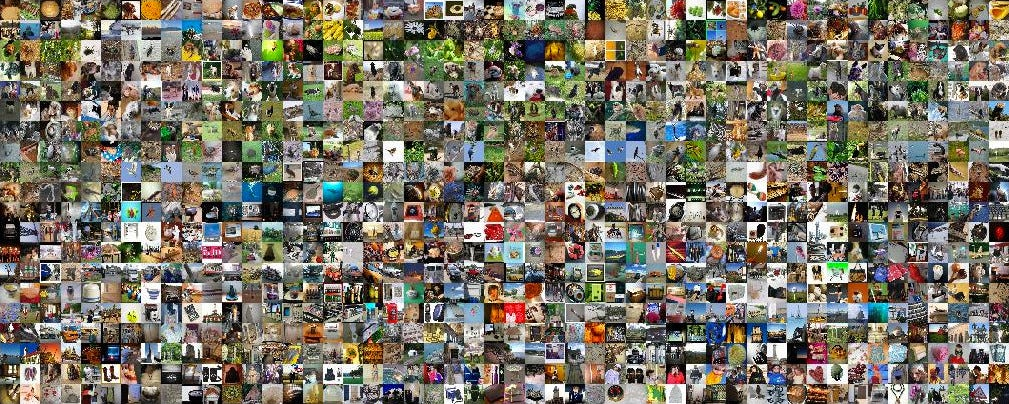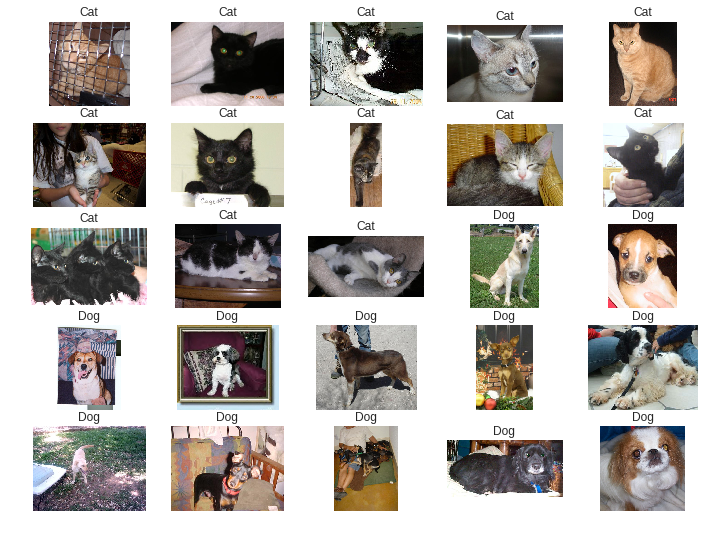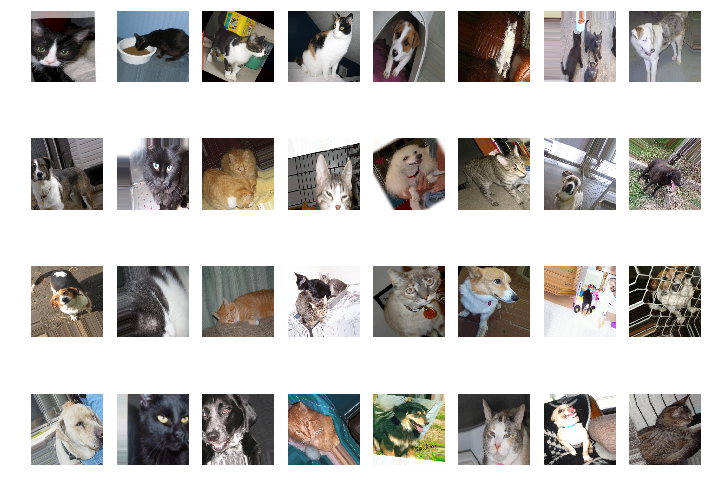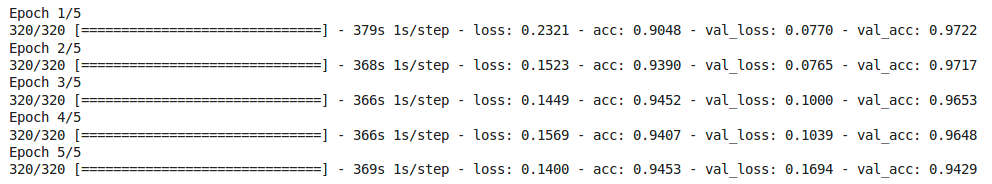# First steps with Transfer Learning for custom image classification with KerasSome ImageNet thumbnails

In a previous post, we covered how to use Keras in Colaboratory to recognize any of the 1000 object categories in the ImageNet visual recognition challenge using the Inception-v3 architecture. But, what happen if we want to predict any other categories that are not in that list?

A practical approach is to use transfer learning — transferring the network weights trained on a previous task like ImageNet to a new task — to adapt a pre-trained deep classifier to our own requirements.

In this post, we are going to introduce transfer learning using Keras to identify custom object categories. To simplify the understanding of the problem we are going to use the cats and dogs dataset. The full code is available as a Colaboratory notebook.

### Why use transfer learning?

It is well known that convolutional networks (CNNs) require significant amounts of data and resources to train. For example, the ImageNet ILSVRC model was trained on 1.2 million images over the period of 2–3 weeks across multiple GPUs.

Transfer learning has become the norm from the work of Razavian et al (2014) because it reduces the training time and data needed to achieve a custom task. It takes a CNN that has been pre-trained (typically ImageNet), removes the last fully-connected layer and replaces it with our custom fully-connected layer, treating the original CNN as a feature extractor for the new dataset. Once replaced the last fully-connected layer we train the classifier for the new dataset.

### Creating the Notebook

To start with custom image classification we just need to access Colaboratory and create a new notebook, following New Notebook > New Python 3 Notebook.

An important step for training it is to select the default hardware CPU to GPU, just following Edit > Notebook settings or Runtime>Change runtime type and select GPU as Hardware accelerator.

Now we can check if we are using the GPU running the following code:

``import tensorflow as tftf.test.gpu_device_name()``

Configured the Notebook we just need to install Keras to be ready to start with transfer learning.

### 1. Data preparation

The first step on every classification problem concerns data preparation. In this case, we will use Kaggle’s Dogs vs Cats dataset, which contains 25,000 images of cats and dogs.

`!wget https://download.microsoft.com/download/3/E/1/3E1C3F21-ECDB-4869-8368-6DEBA77B919F/kagglecatsanddogs_3367a.zip!unzip kagglecatsanddogs_3367a.zip!mv PetImages train`Sample images from Kaggle’s Cat vs Dog dataset

Downloaded the dataset, we need to split some data for testing and validation, moving images to the train and test folders. We use the `train_test_split()` function from scikit-learn to build these two sets of data. Thus, we create a structure with training and testing data, and a directory for each target class. This is the common folder structure to use for training a custom image classifier — with any number of classes — with Keras.

`train/  Cat/  Dog/test/  Cat/  Dog/`

### 2. Model customization

Prepared the dataset, we can define our network. We are going to instantiate the `InceptionV3` network from the `keras.applications` module, but using the flag `include_top=False` to load the model and their weights but leaving out the last fully connected layer, since that is specific to the ImageNet competition.

`base_model = InceptionV3(weights='imagenet', include_top=False)`

Then we add our custom classification layer, preserving the original Inception-v3 architecture but adapting the output to our number of classes. We use a `GlobalAveragePooling2D` preceding the fully-connected `Dense` layer of `2` outputs.

`CLASSES = 2`
`x = base_model.outputx = GlobalAveragePooling2D(name='avg_pool')(x)x = Dropout(0.4)(x)predictions = Dense(CLASSES, activation='softmax')(x)model = Model(inputs=base_model.input, outputs=predictions)`

Now we need to freeze all our `base_model` layers and train the last ones. An additional step can be performed after this initial training un-freezing some lower convolutional layers and retraining the classifier with a lower learning rate. This fine-tuning step increases the network accuracy but must be carefully carried out to avoid overfitting.

`for layer in base_model.layers:    layer.trainable = False`

Finally, we compile the model selecting the optimizer, the loss function, and the metric. In this case we are going to use a RMSProp optimizer with the default learning rate of 0.001, and a `categorical_crossentropy` — used in multiclass classification tasks — as loss function.

`model.compile(optimizer='rmsprop',              loss='categorical_crossentropy',              metrics=['accuracy'])`

### 3. Data augmentation

Data augmentation is a common step used for increasing the dataset size and the model generalizability. Essentially, it is the process of artificially increasing the size of a dataset via transformations — rotation, flipping, cropping, stretching, lens correction, etc — .

Keras provides the class `ImageDataGenerator()` for data augmentation. This class can be parametrized to implement several transformations, and our task will be decide which transformations make sense for our data. Images will be directly taken form our defined folder structure using the method `flow_from_directory()`.Batch output sample from the ImageDataGenerator class

Preparing our data generators, we need to note the importance of the preprocessing step to adapt the input image data values to the network expected range values. This is set using the `preprocess_input` from the `keras.applications.inception_v3` module.

`from keras.applications.inception_v3 import preprocess_input`
`train_datagen = ImageDataGenerator(    preprocessing_function=preprocess_input,    rotation_range=40,    width_shift_range=0.2,    height_shift_range=0.2,    shear_range=0.2,    zoom_range=0.2,    horizontal_flip=True,    fill_mode='nearest')`

Then, we configure the range parameters for `rotation`, `shifting`, `shearing`, `zooming`, and `flipping` transformations.

### 4. Transfer learning

Finally, we can train our custom classifier using the `fit_generator` method for transfer learning. In this example, it is going to take just a few minutes and five epochs to converge with a good accuracy.

`EPOCHS = 5BATCH_SIZE = 32STEPS_PER_EPOCH = 320VALIDATION_STEPS = 64`
`MODEL_FILE = 'filename.model'`
`history = model.fit_generator(    train_generator,    epochs=EPOCHS,    steps_per_epoch=STEPS_PER_EPOCH,    validation_data=validation_generator,    validation_steps=VALIDATION_STEPS)  model.save(MODEL_FILE)`

It is important to note that we have defined three values: `EPOCHS`, `STEPS_PER_EPOCH`, and `BATCH_SIZE`. These values appear because we cannot pass all the data to the computer at once (due to memory limitations). So, to overcome this problem we need to divide the dataset into smaller pieces (batches) and give it to our computer one by one, updating the weights of the neural network at the end of every step (iteration) to fit it to the data given.

We have defined a typical `BATCH_SIZE` of 32 images, which is the number of training examples present in a single iteration or step. And 320 `STEPS_PER_EPOCH` as the number of iterations or batches needed to complete one epoch.Metrics log after 5 epochs

Learning is an iterative process, and one epoch is when an entire dataset is passed through the neural network. The number of epochs controls weight fitting, from underfitting to optimal to overfitting, and it must be carefully selected and monitored.

### 5. Prediction

Now that we have trained the model and saved it in `MODEL_FILE`, we can use it to predict the class of an image file — if there is a cat or a dog in an image— . Even after only 5 epochs, the performance of this model is pretty high, with an accuracy over 94%.

`import numpy as npfrom keras.preprocessing import imagefrom keras.models import load_model`
`def predict(model, img):    x = image.img_to_array(img)    x = np.expand_dims(x, axis=0)    x = preprocess_input(x)    preds = model.predict(x)    return preds`
`img = image.load_img('test/Dog/110.jpg', target_size=(HEIGHT, WIDTH))preds = predict(load_model(MODEL_FILE), img)`

For instance, we can see bellow some results returned for this model:

### Conclusions

This introduction to transfer learning presents the steps required to adapt a CNN for custom image classification. For simplicity, it uses the cats and dogs dataset, and omits several code. The full code is available as a Colaboratory notebook.

In a next article, we are going to apply transfer learning for a more practical problem of multiclass image classification.